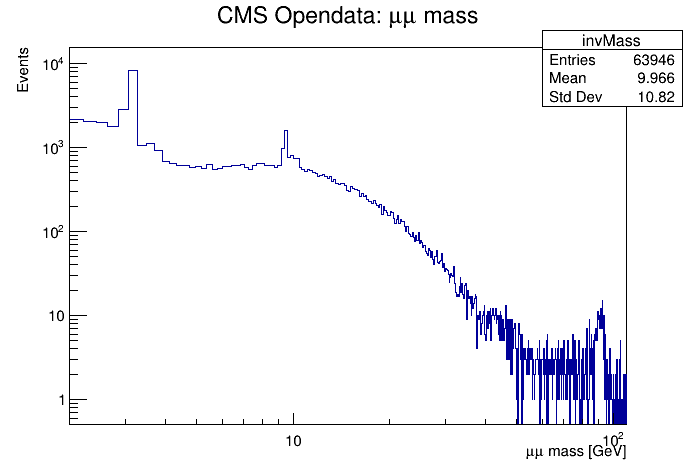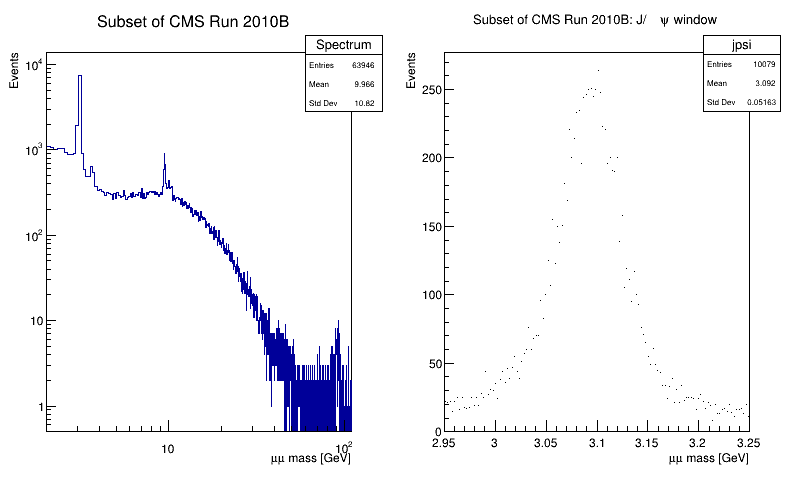# Df 0 1 4_ C S V Data Source¶

Process a CSV file with RDataFrame and the CSV data source.

This tutorial illustrates how use the RDataFrame in combination with a RDataSource. In this case we use a RCsvDS. This data source allows to read a CSV file from a RDataFrame. As a result of running this tutorial, we will produce plots of the dimuon spectrum starting from a subset of the CMS collision events of Run2010B. Dataset Reference: McCauley, T. (2014). Dimuon event information derived from the Run2010B public Mu dataset. CERN Open Data Portal. DOI: 10.7483/OPENDATA.CMS.CB8H.MFFA.

Author: Enric Tejedor (CERN)
This notebook tutorial was automatically generated with ROOTBOOK-izer from the macro found in the ROOT repository on Thursday, June 24, 2021 at 07:13 AM.

Let's first create a rdf that will read from the csv file. The types of the columns will be automatically inferred.

In :
auto fileNameUrl = "http://root.cern.ch/files/tutorials/df014_CsvDataSource_MuRun2010B.csv";
auto fileName = "df014_CsvDataSource_MuRun2010B_cpp.csv";
if(gSystem->AccessPathName(fileName))
TFile::Cp(fileNameUrl, fileName);
auto df = ROOT::RDF::MakeCsvDataFrame(fileName);

[TFile::Cp] Total 14.46 MB	|====================| 100.00 % [81.4 MB/s]


Now we will apply a first filter based on two columns of the csv, and we will define a new column that will contain the invariant mass. Note how the new invariant mass column is defined from several other columns that already existed in the CSV file.

In :
auto filteredEvents =
df.Filter("Q1 * Q2 == -1")
.Define("m", "sqrt(pow(E1 + E2, 2) - (pow(px1 + px2, 2) + pow(py1 + py2, 2) + pow(pz1 + pz2, 2)))");


Next we create a histogram to hold the invariant mass values and we draw it.

In :
auto invMass =
filteredEvents.Histo1D({"invMass", "CMS Opendata: #mu#mu mass;#mu#mu mass [GeV];Events", 512, 2, 110}, "m");

auto c = new TCanvas();
c->SetLogx();
c->SetLogy();
invMass->DrawClone();


We will now produce a plot also for the j/psi particle. we will plot on the same canvas the full spectrum and the zoom in the J/psi particle. First we will create the full spectrum histogram from the invariant mass column, using a different histogram model than before.

In :
auto fullSpectrum =
filteredEvents.Histo1D({"Spectrum", "Subset of CMS Run 2010B;#mu#mu mass [GeV];Events", 1024, 2, 110}, "m");


Next we will create the histogram for the j/psi particle, applying first the corresponding cut.

In :
double jpsiLow = 2.95;
double jpsiHigh = 3.25;
auto jpsiCut = [jpsiLow, jpsiHigh](double m) { return m < jpsiHigh && m > jpsiLow; };
auto jpsi =
filteredEvents.Filter(jpsiCut, {"m"})
.Histo1D({"jpsi", "Subset of CMS Run 2010B: J/#psi window;#mu#mu mass [GeV];Events", 128, jpsiLow, jpsiHigh},
"m");

input_line_76:4:1: warning: captures will be by reference, not copy
auto jpsiCut = [jpsiLow, jpsiHigh](double m) { return m < jpsiHigh && m > jpsiLow; };
^


Finally we draw the two histograms side by side.

In :
auto dualCanvas = new TCanvas("DualCanvas", "DualCanvas", 800, 512);
dualCanvas->Divide(2, 1);
auto leftPad = dualCanvas->cd(1);
leftPad->SetLogx();
leftPad->SetLogy();
fullSpectrum->DrawClone("Hist");
dualCanvas->cd(2);
jpsi->DrawClone("HistP");

return 0;


Draw all canvases

In :
gROOT->GetListOfCanvases()->Draw()